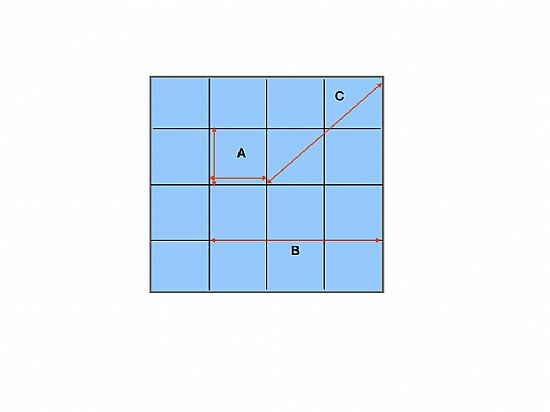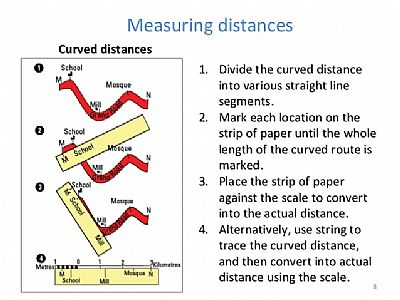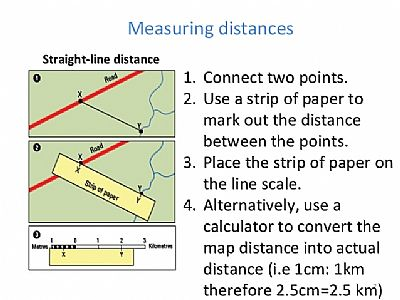## Measurement of Distance

Introduction

The measurement of distance is one of the most important uses of a map. However it is essential to note that distances scaled directly from a map are horizontal distances which take into no account of slopes. So when estimating the duration of a proposed journey proper allowance must be mad for the shape of the ground.

Straight Line Distances

The following methods of measuring straight line distances are given in ascending order accuracy.

• Use of Grid Lines. Most maps at scales larger than 1:1000,000 carry grid lines which are a fixed distance apart. A quick estimate of the distance between two points can be obtained by counting the grid squares between them. This method is ok for distances in the north-south or east-west directions but significant errors accumalate in the diagonal directions.

As you an see in this simple diagram of one grid square of a 1,000 meters sq the diagonal distance measures 1400 meters• Position A: One gird square measures 1000x1000 metres or 1 kilometre square.
• Position B: Distance horizontal or vertical in this case three grid squares gives an estimated distance of 3 kilometres'
• Position C: Diagonally the distance across two grid squares would be an estimated distance of 2.8 Kilometres. This is because the distance in on grid square equates to 1400 metres or 1.4 kilometres.

Speed Time and Distance

When planning any movement it may be advisable to calculate an estimated time of arrival (ETA) or if the arrival time is pre-determined it is neccessary to calculate and estimated time of departure (ETD) which will ensure arrival at appointed time. The variables are thus Speed, Time and Distance.

Speed = Distance over Time

Time = Distance over Speed

Distance = Speed x Time

Knowing how long a particular walk is going to take you is essential. So if you where going to walk to your destination which is 18 km away where you are going to get picked up at say 1630 hrs.and you walking speed is 5 kph.

Elapsed time for the journey = 18 over 5 hrs = 3.6hrs = 3hrs 36min

Latest departure time = Arrival time - elapsed time

=1630hrs - 3hrs 36 min

=1254 hrs

A walking speed of of 5kph represents 12 min per km.

Measuring distances on roads paths and tracks.

To measure a distance which is not straight, eg, along a road, path track or river, stream, consider the feature as anumber of straight or nearly straight sections. Lay a piece of paper along the first section, and mark it with a tick at the starting point and another at the end of the first section. Then pivot the paper about second tick until it lies along the second section. Keep repeating this until you come to the end.

The total distance along the road is then recorded as a straight line on the paper. It can then be placed along the relevant scale at the bottom of the map to find out the distance